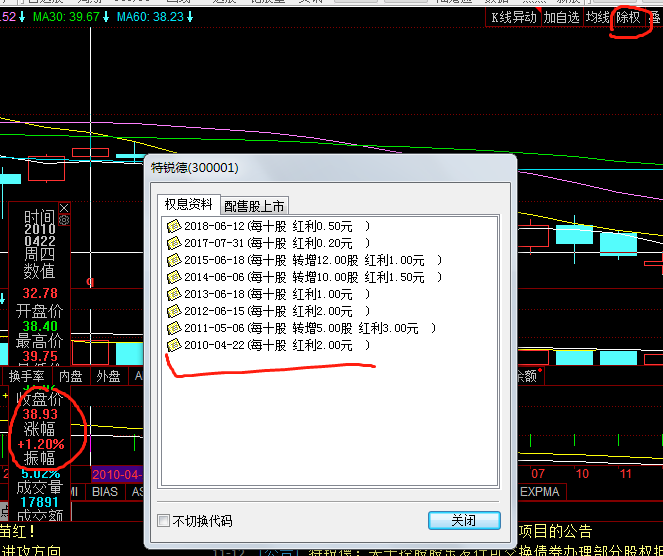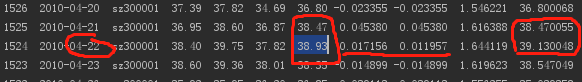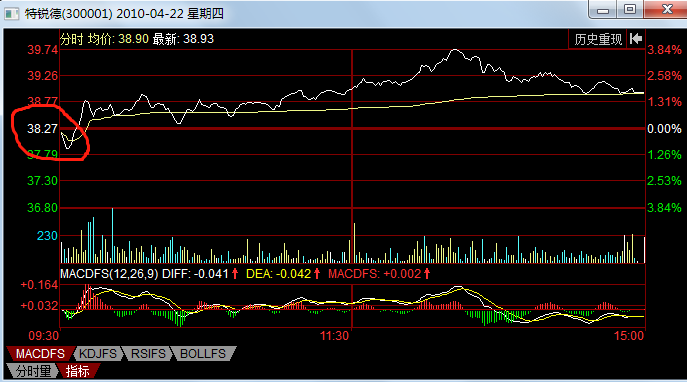# 除权、除息、复权、填权、填息、贴权、贴息、含权、含息、前复权、后复权到底什么区别（MD终于明白了&用图解释）

• ## 除权、除息、复权、填权、填息、贴权、贴息、含权、含息、前复权、后复权到底谁是谁（MD终于明白了）

$价格四舍五入到0.01元$

$除息价=股息登记日的收盘价-每股所分红利现金额$

$送股除权价=\cfrac{股权登记日的收盘价}{（1+每股送红股数）}$

$配股除权价=\cfrac{（股权登记日收盘价+配股价*每股配股数）}{（1+每股配股数）}$

$送股除权除息价 = \cfrac{股权登记日收盘价-每股派息额}{1+每股送股数}$

$配股除权除息价 = \cfrac{股权登记日收盘价-每股派息额+配股价*每股配股数}{1+每股配股数}$

$送、配股除权除息价 = \cfrac{股权登记日收盘价-每股派息额+配股价*每股配股数}{1+每股送股数+每股配股数}$

$复权后价格＝复权前价格*(1＋流通股份变动比例) + 现金红利 - 配(新)股价格*流通股份变动比例$

$后复权价 = \cfrac{股权登记日收盘价-每股派息额+配股价*每股配股数}{1+每股送股数+每股配股数}$

• ## 备注

$复权因子 = （真是涨跌幅 + 1）.cumprod(累乘函数)$

$股票上市初始价格*复权因子=股票上市以来的累计涨幅。$2010.4.22是该股票第一次除息，10股送2元，第2张图可以看到2010.4.21收盘价38.47，则除权除息日得到除权价为38.47-0.2=38.27，即第三张图显示的开盘价（参考第一张右上角，此时是同花顺除权条件下的显示），由第一张图看到2010.4.22收盘价38.93，对于当天(38.93-38.27)/38.27=0.0172，而第一张图中显示涨幅是1.2%。这个1.2%是这么来的：(38.93-38.47)/38.47=0.0119。

.
.
.
2018-11-21 17:1823修改于德州乐陵